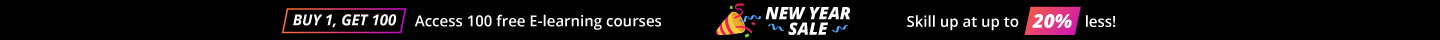# Machine Learning Interview Questions Data Science

Ready to face your next Machine Learning interview? Be interview-ready with this list of Machine Learning interview questions and answers, carefully curated by industry experts. Be ready to answer different questions like CRISP-DM, difference between univariate and bivariate analysis, chi-square test, difference between Type 1 and Type 2 Error, Bias-Variance trade-off. We have gathered a set of interview questions for machine learning that will help you become a machine learning engineer, data engineer.

• 4.6 Rating
• 40 Question(s)

## Intermediate

CRISP-DM stands for Cross Industry Standard Process for Data Mining. It is a methodology for data science programs. It has the following phases:

1. Business understanding – (Typical tasks are: Determine business objective, Assess Situation, Determine Data mining goals, project plan)
2. Data understanding – (Collect initial data, Describe data, Explore Data, Verify Data Quality)
3. Data preparation – (Select data, Clean data, Construct data, Integrate data, Format data)
4. Modelling or Model development – (Select Modelling techniques, Generate test design, Build model, Assess model)
5. Model evaluation – (Evaluate results, Review process, Determine next steps)
6. Deployment – (Plan deployment, Plan monitoring & maintenance, Product final report & Review Project)

Some phases are iterative in nature and any data science project or program which is end to end typically follows this methodology.

Below is a diagrammatic view for better understanding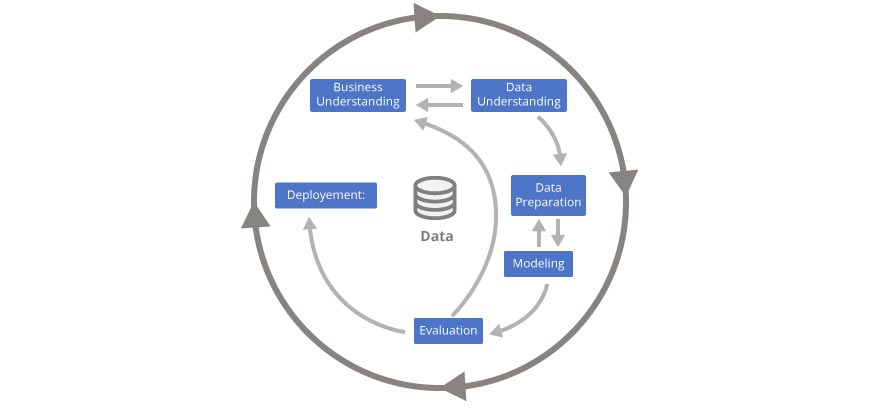In univariate analysis, variables are explored one by one. Method to perform univariate analysis will depend on whether the variable type is categorical or continuous.

In the case of continuous variables, we need to understand the central tendency and spread of the variable. For example- central tendency – mean, median, mode, max, min, etc.; a measure of dispersion – range, quartile, IQR, variance, standard deviation, skewness, kurtosis etc; visualization methods – histogram, boxplot etc.

Univariate analysis is also used to highlight missing and outlier values.

The relationship between two variables can be determined using bivariate analysis. How the two variables are associated and/or dis-associated are looked into considering the significance level of comparison. Typically bivariate analysis can be performed for:

1. two categorical variables
2. categorical and continuous variables
3. two continuous variables

Different approaches/methods need to be used to handle the above scenarios. Scatter plot can be used irrespective of whether a relationship is linear or nonlinear. In order to figure out how loosely or tightly both variables are correlated, correlation can be performed where the correlation values indicate from -1 to 1. If the value indicates 0, then there is no correlation between the two variables. If it is -1, then there is a perfect -ve correlation and if it is a +1 then it is a perfect +ve correlation.

When we want to find out the statistical significance between two variables, then the chi-square test is used to understand the deviation between observed and expected frequency and divided by the expected frequency.

• Probability of 0: It indicates that both categorical  variables are dependent
• Probability of 1: It shows that both variables are independent.
• Probability less than 0.05: It indicates that the relationship between the variables is significant at 95% confidence. The chi-square test statistic for a test of independence of two categorical variables is found by:
• Square of X = Sum[Square of (O - E) / E]
• Where O – Observed frequency
• E – Expected frequency under the null hypothesis

We use this between two Categorical variables.

When variables are categorical and continuous, and there are “many samples”, then we should not use the t-test. If sample size n>=30, then we can go for z-test. When there are too many samples and the mean/average of multiple groups are to be compared, then ANOVA can be chosen.

When we don’t have many samples and variance is unknown, then we will use the t-test. In a t-test, the expectation is that the sample size is smaller. Typical n<30, where n is the number of observations or sample size.

The t-test and z-test can be defined as follows. There is a very subtle difference between the two. z-test is used for n>=30 and t-test is used for n<30 scenarios mostly.

t-test = (x-bar - mu) / (sd / sqrt(n))

• where x-bar = sample average or sample mean of x
• mu = population average or population mean
• sd = standard deviation of a sample
• n = number of observations, which is sample size

z-test = (x-bar - mu) / (sigma / sqrt(n))

• where x-bar = sample average or sample mean of x
• mu = population average or population mean
• sigma = standard deviation of a population
• n = number of observations, which is sample size

ANOVA is an analysis of variance. For example, let’s say we are talking about 3 groups.

Class 1Class 2Class 3
893
624
563
825
674
1054
626
384
545
793

Figure ANOVA

In the “Figure ANOVA” above, we can consider ANOVA for analysis as there are more than 2 sample groups. i.e. 3 groups of samples. There can be many rows in each class. We have considered only 10 each for simple understanding.

Class GroupCountSumAverageVariance
Class 110646.43.82
Class 210545.48.04
Class 310414.10.99

Missing data in the training data set can reduce the power/fit of a model or can lead to a biased model because we have not analyzed the behavior and relationship with other variables correctly. It can lead to incorrect prediction or classification. Below is a simple example to illustrate this.

Name
Weight
Gender
Play Golf or Not
AA55MYes
BB62FYes
CC58FNo
DD54
No
EE54MNo
FF66FYes
GG56
Yes
HH56MYes

Figure 1

Gender
# Count
# Play Golf
% Play Golf
F3266.67%
M3266.67%
Missing/Blank2150%

Figure 2

Please note the missing values in the table shown above: in figure1, we have not treated missing values for our analysis in Figure 2. The inference from this data set is that the chances of playing golf by females and males are similar.

On the other hand, if you look at Figure. 4, which shows data after treatment of missing values (based on gender), we can see that females have higher chances of playing cricket compared to males.

Name
Weight
Gender
Play Golf or Not
AA55MYes
BB62FYes
CC58FNo
DD54MNo
EE54MNo
FF66FYes
GG56MYes
HH56MYes

Figure 3

Gender
# Count
# Play Golf
% Play Golf
F3266.67%
M5360%

Figure 4

Below are different types of missing values can occur while the data collection process.

1. Missing values completely at random - If the probability of missing variable is the same across all observations, then it falls into this category. For example students determine that they will declare their preference whether to go to a cultural festival or not after tossing a fair coin. If a head occurs, then will declare that they will either go or do not decide to go and vice versa. Each observation has an equal chance of missing value whether to go or not go.
2. Missing values at random - This is different than “a” mentioned above. If the variable is missing at random and the missing ratio differs for different values of input variables, then this scenario occurs. For example: in a fair coin example setup, we have information of a set of people in a locality about their demographics, age, sex, locality type - busy/very busy/moderate busy, etc and if a female has a higher missing value of other parameters compared to male.
3. The missing value that depends on unobserved predictors - This case is possible when missing values are not completely at random. The phenomenon is based on unobserved input variable. Let’s say for example there is a mathematics examination and because of the complex level of examination, the expectation is that there will be fewer students who will go and appear the exam. Out of 100 students, 30 do not appear because of the “complexity level” of examination. This type of missing value is not at random. Instead, this is due to “complexity level” unless this parameter is not taken into account as a cause already.
4. The missing value which depends on missing value itself - This is a scenario when the probability of a missing value is correlated with the missing value itself. For example Students with higher or lower marks in graded exam in one subject are likely to appear/disappear in competitive exam for the same subject for another purpose/competition.

When a particular variable is missing in an observation or row, then we delete an entire row. This is called List wise deletion.

When the analysis is performed with all cases of a variable and then only those variable instances are deleted and not the entire row. This is called Pairwise deletion. This works like a correlation matrix.

Generally, pairwise deletion is preferred over listwise deletion as listwise deletion removes the entire row for a particular missing variable.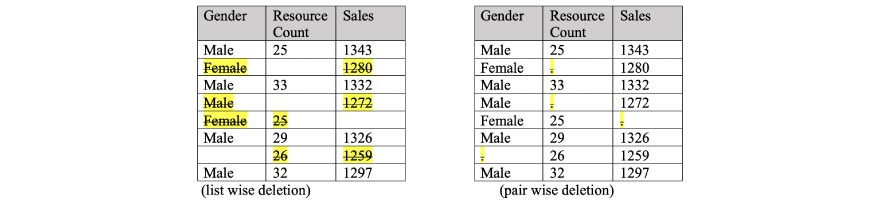It is one of the methods to treat missing values other than direct deletion, imputation using a mean/median/mode value, etc. In kNN imputation, the missing values of an attribute are imputed using the given number of attributes that are most similar to the attribute whose values are missing. The similarity of two attributes is determined using a distance function. Pros and Cons are described below.

Pros
Cons
• It can predict both qualitative & quantitative attributes.
• Creation of predictive model for each attribute with missing data is not required.
• Attributes with multiple missing values can be easily treated.
• Correlation structure of the data is taken into consideration.

• It is very time-consuming in analysing large database. It searches through all the dataset looking for the most similar instances. Hence complex and takes time.
• Choice of k-value is very critical. Higher value of k would include attributes which are significantly different from what we need whereas lower value of k implies missing out of significant attributes

Outliers can have a significant impact based on the results of the data analysis and statistical modeling. These are as follows:

• Outliers can decrease normality as they are non-randomly distributed
• Error variance increases with a relative comparison and that provides an incorrect estimate of the overall population.
• Power of statistical tests are also reduced because of the impact in standard deviation.
• ANOVA, different relevant statistical model assumptions are impacted.

Here is an example with a sample dataset.

Without Outlier
With Outlier

Dataset: 1,1,2,2,2,2,3,3,3,4,4

Mean = 2.45

Median = 2.00

Mode = 2.00

Standard deviation = 1.035

Dataset: 1,1,2,2,2,2,3,3,3,4,4,200

Mean = 18.91

Median = 2.50

Mode = 2.00

Standard deviation = 57.03

If we look at above, inclusion of an outlier shows huge difference in mean / average and standard deviation parameters.

There are various methods.

1. The most common method is to use visualization using box-plots, histograms and scatter plots to detect outliers.
2. Another way - Any value, which is beyond the range of -1.5 x IQR to 1.5 x IQR (where IQR stands for Inter-Quartile Range).
3. Use capping methods. Any value which out of range of 5th and 95th percentile can be considered an outlier.

Others could be as follows: Data points, three or more standard deviations away from the mean are considered as outlier.

There could be many assumptions. Five of them are described below:

1. The regression model is linear in parameters.
2. The mean of residuals is zero.
3. Homoscedasticity of residuals or equal variance.
4. No autocorrelations of residuals.
5. No perfect multicollinearity i.e. no perfect linear relationship between explanatory variables

A stationary time series has the following characteristics:

1. Mean or average is constant over time
2. Variance is constant over time
3. Autocorrelation is constant over time
4. Seasonality component may not have much impact over time

This type of time series is typically easy to predict as there not much variations expected in the pattern and trend.

Autocorrelation and partial autocorrelation are a type of measures of association between current time series and past time series values. Both of these provide an indication that older time series values are more useful in predicting future values.

Autocorrelation is the correlation of a Time Series with lags of itself. This is a significant metric because:

1. It is able to demonstrate the difference between a past state of time series observations and current state of time series observations and how much of lag impact. In below diagram time series of “Air Passengers” dataset is considered and ACF plot is drawn. As ACF lines are consistently outside of the dotted line, it indicates a significant correlation of lags in the series.
2. It also provides the aspect that the series is stationary or not. Autocorrelation plot is also called correlogram. If there is a stationary element, then correlogram will fall to zero, else if there is no stationary element, then correlogram will fall gradually slowly.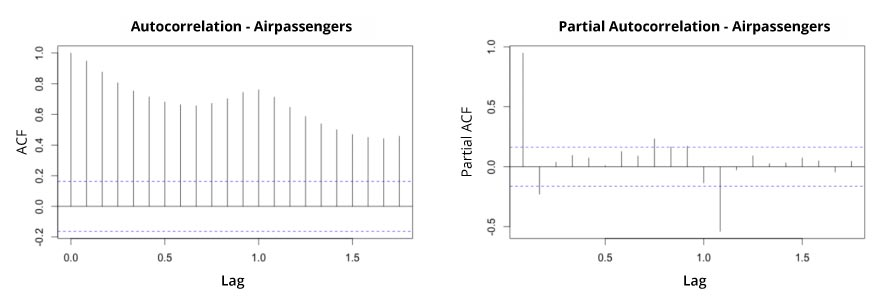While comparing current time series steps to that of prior time series steps, there can be direct and indirect correlations. The indirect correlations are a linear function of correlation of the observation. There could be intervening time series steps. PACF or Partial autocorrelation tries to remove the effect of correlation due to shorter lags.

Both ACF and PACF are useful while trying to understand which model approach could be a relevant and better fit for a prediction solution.

Linear regression can be used to model the Time Series data with linear indices (Ex: 1, 2,...n). The resulting model’s residuals are a representation of the time series devoid of the trend.

In case, if some trend is left over to be seen in the residuals (like what it seems to be with ‘Figure1’ with myData below as an example), then you might wish to add few predictors to the lm() call (like a forecast:: seasonal dummy, forecast::Fourier or may be a lag of the series itself), until the trend is filtered.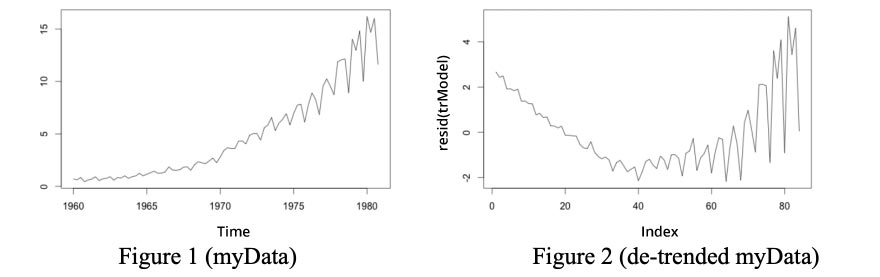Code snippet:

trModel <- lm(myData ~ c(1:length(myData)))
plot(resid(trModel), type="l")  # resid(trModel) contains the de-trended series

We can use the Augmented Dickey-Fuller Test (adf test) to test “stationary” aspect. A p-Value of less than 0.05 in adf.test() indicates that it is stationary.

Illustrative code snippet:

library(tseries)
adf.test(myData) # p-value < 0.05 indicates the TS is stationary kpss.test(myData)

It is a statistical test used to compare two related and matched samples. If a population can not be assumed to be normally distributed, then this test may be useful with the assumption that data are paired and from the same population. Each data pair is chosen randomly. It tries to compare between sample median and hypothetical median.

The boxplot below in R with the “air quality” sample data demonstrates the interpretation of the analysis using this test.

• boxplot(Ozone ~ Month, data = airquality)
• wilcox.test(Ozone ~ Month, data = airquality, subset = Month %in% c(5, 8))
• Wilcoxon rank sum test with continuity correction
• data: Ozone by Month
• W = 127.5, p-value = 0.0001208
• alternative hypothesis: true location shift is not equal to 0 Interpretation is this:
• If p-Value < 0.05, reject the null hypothesis and accept the alternative mentioned in your R code’s output.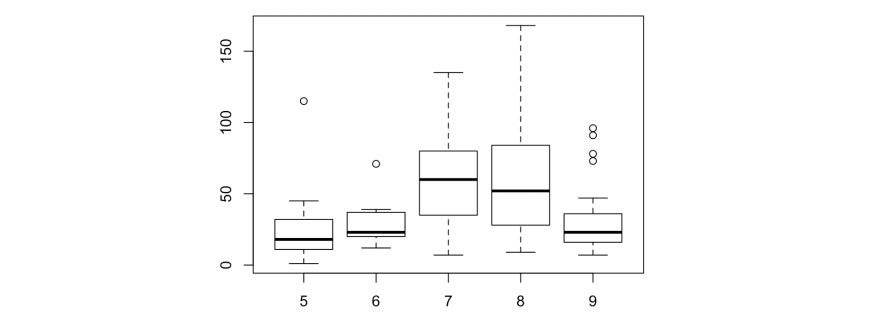Kolmogorov-Smirnov test is used to check whether 2 samples follow the same distribution.

• x <- rnorm(50)
• y <- runif(50)
• ks.test(x, y)

Two-sample Kolmogorov-Smirnov test

data: x and y

D = 0.52, p-value = 1.581e-06

alternative hypothesis: two-sided

• x <- rnorm(50)
• y <- rnorm(50)
• ks.test(x, y)

Two-sample Kolmogorov-Smirnov test

data: x and y

D = 0.1, p-value = 0.9667

alternative hypothesis: two-sided

If p-Value < 0.05 (significance level), we reject the null hypothesis that they are drawn from the same distribution. In other words, p < 0.05 implies x and y from different distributions.

Jitter plot is used for correlation. It provides pretty much all points which scatter plots typically do not show up.

We consider mpg dataset with city mileage (cty) and highway mileage (hwy). The original data has 234 data points but a typical scatter plot seems to display fewer points.

This is because there are many overlapping points appearing as a single dot. The fact that both cty and hwy are integers in the source dataset made it all the more convenient to hide this detail.

• load package and data library(ggplot2)

data(mpg, package="ggplot2") theme_set(theme_bw())

g <- ggplot(mpg, aes(cty, hwy))

• Scatterplot

g + geom_point() +

geom_smooth(method="lm", se=F) +

labs(subtitle="mpg: city vs highway mileage",

y="hwy",

x="cty",

title="Scatterplot with overlapping points",

caption="Source: midwest")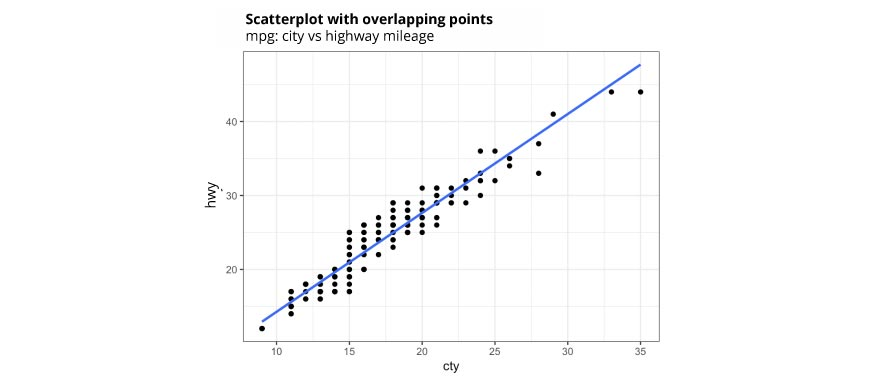Now we can handle this with a Jitter plot.

We can make a jitter plot with jitter_geom(). As the name suggests, the overlapping points are randomly jittered around its original position based on a threshold controlled by

the width argument.

• load package and data library(ggplot2)

data(mpg, package="ggplot2")

• Jitter plot

theme_set(theme_bw()) # pre-set the bw theme.

g <- ggplot(mpg, aes(cty, hwy))

g + geom_jitter(width = .5, size=1) + labs(subtitle="mpg: city vs highway mileage",

y="hwy",

x="cty",

title="Jittered Points")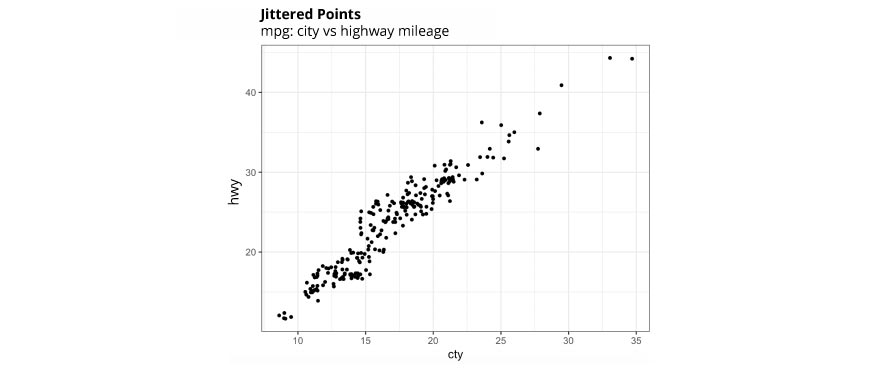There are three types of error in any machine learning approach. They are a biased error, variance error, and irreducible error. Generally, the focus is to look at striking a balance between bias and variance and reducing those errors in the model so that accuracy can be improved.

Low Bias - indicates fewer assumptions about the form of the target variable or function. In this case, when we test on new data, it does not give expected results and accuracy can be compromised.

High variance - indicates large changes to the estimate of target variable or target function with changes to the training data.

It is always tricky to handle scenario to balance between these two as increasing the bias will decrease the variance and increasing the variance will decrease the bias. Hence approach that can be followed are as follows:

1. Look at the dataset in hand and contextual information to suggest which way to look at for a better prediction performance
2. Typically kNN and SVM algorithms can be looked at depending on what problem we are solving and how tweaks can be performed in order to manage this effect

This can be described in the below table.

kNN
k-means clustering
This is supervised machine learning
This is unsupervised machine learning
This is used for classification and regression problems.
As the name suggests, it is a clustering algorithm.
This is based on feature similarity.
This divides objects or set of data points into clusters.
No such mechanism here.
Typically k=3 or based on elbow diagram, k value can be determined

For example, let’s consider a dataset of football players, their positions, their measurements, etc. We want to assign a position to these players in a new dataset which is unseen by the model which is learned using earlier training data. We may use kNN algorithm since there are measurements, but no positions are known. At the same time, let’s say we have another scenario where we have a dataset of these football players who are to be grouped into some specific groups based on some similarity between them. In this case, k-means could be used. So, both of these are context specific to the problem we are trying to solve.

In a regression problem, we expect that when we define a solution or mathematical formula, it should explain all possible values or assumption is that most data points should get closer to the line if it is a linear regression.

R square is also known as “goodness of fit”. The higher the value of R square, the better it is. R square explains the amount to which input variables explain the variation of the target variable or predicted variable. If R square is 0.75, then it indicates that 75% of the variation in the target variable is explained by input variables. So higher the R-square value, better the explainability of variation in target, hence better the model performance.

Now the problem arises, where we add more input variables. The value of R-square keeps increasing. If additional variables do not have an influence in determining the variation of the target variable, then it is a problem and higher R-square value, in this case, is misleading. This is where the adjusted R square is being used. The Adjusted R square is an updated version of R square. It penalizes if the addition of more input variables does not improve the existing model and can’t explain the variation in target effectively.

So if we are adding more input variables, we need to ensure they influence target variable, else the gap between R-square and Adjusted R-square will increase. If there is only one input variable both value will be the same. If there are multiple input variables, it is suggested to consider Adjusted R-square value for the goodness of fit.

Tolerance is defined as 1/VIF where VIF stands for Variation Inflation Factor. VIF as the name suggests indicates the inflation in variation. It is a parameter that detects multicollinearity between variables. Based on VIF values, we can determine whether to remove or include all variables without comprising the Adjusted R-square value. Hence 1/VIF or Tolerance can be used to gauge which all parameters to be considered in the model to have a better performance.

Type I error is committed when the null hypothesis is true and we reject it, also known as a ‘False Positive’. Type II error is committed when the null hypothesis is false and we accept it, also known as ‘False Negative’.

In the context of the confusion matrix, we can say Type I error occurs when we classify a value as positive (1) when it is actually negative (0). Type II error occurs when we classify a value as negative (0) when it is actually positive(1).

Logistic Regression models can be evaluated as follows:

1. First and foremost key parameter for evaluation is AUC-ROC curve. This is the Area under Curve. The confusion matrix can be built or generated based on actual and predicted values from the model solution. Based on that, the AUC-ROC curve can be plotted to see the model performance. ROC stands for Receiver Operating Characteristic. For an ideal model, the perfect True positive rate score will be 1 and False Positive rate will be 0. The more inclined the ROC curve towards 1, the better it is.
2. Secondly, another important metrics is AIC which stands for Akaike Information Criteria. This is related to the Adjusted R square value. When we look at R square and Adjusted R square, we understand that when there are more input variables being added without improving the variation explanation of target variable, then metric such as Adjusted R square penalizes if we add input variables just for the sake of adding and no value in terms of model performance. Hence in such cases, Adjusted R square is a better interpretation compared to R square and hence it is followed. AIC value is dependent on Adjusted R square. Hence, AIC is the goodness of fit and it penalizes if more variables are added to a model without adding value.
3. Null deviance and Residual deviance are other metrics which are important to evaluate a logistic regression model. Both should be low which will indicate the model is better.

Machine learning can be of types - supervised, unsupervised and others such as semi-supervised, reinforcement learning, etc.

When we look at how to choose which algorithm to select, it depends on input data type primarily and what are we trying to accomplish out of it.

1. If the target variable is continuous, then we will use regression algorithms (which are part of supervised learning). e.g. Simple Linear Regression, Multiple Linear Regression, etc.
2. If the target variable is categorical, then we will use classification algorithms (this is also part of supervised learning). e.g. Logistic Regression, Random Forest, Decision Trees, kNN, Neural Network, Support Vector Machine, Naive Bayes, etc.
3. If the target variable is not available, then we will use any of the unsupervised learning such as Clustering or Association or Recommendation Algorithms.

Other types of machine learning also used in different scenarios.

Generative, Graph-based and Heuristic approaches are part of semi-supervised learning while reinforcement learning can be active and passive categories.

This is how different machine learning algorithms, methods, approaches can be used at different scenarios at a high level.

Mathematically the error emerging from any model can be broken down into 3 major components.

Error(X) = Square(Bias) + Variance + Irreducible Error

It is important to handle or address the bias error and variance error which is in control. We can’t do much for irreducible error.

• Low Bias - indicates fewer assumptions about the form of the target variable or function. In this case, when we test on new data, it does not give expected results and accuracy can be compromised. High Bias indicates high assumptions in a similar context.
• High variance - indicates large changes to the estimate of target variable or target function with changes to the training data. Low variance indicates smaller changes to the estimate of the target variable or target function in a similar context.

When we are trying to build a model with greater accuracy, for better performance of the model, it is critical to strike a balance between bias and variance so that errors can be minimized and the gap between actual and predicted outcomes can be reduced.

Hence balance between Bias and Variance needs to be maintained.

OLS stands for Ordinary Least Squares. OLS is a line or estimate which minimizes the error. The sum squared of errors is considered here. Error is the difference between the observed value and its corresponding predicted value. This is typically in a linear regression model scenario.

MLE stands for maximum Likelihood Estimate. MLE is an approach for estimating parameters of a statistical model. Here random error is assumed to follow a distribution, e.g. normal distribution.

MLE is more to select a parameter that can maximize the likelihood or log-likelihood (when we try to normalize based on data values). OLS considers the parameter value that minimizes the error of the model.

There are various key metrics used for evaluation of a logistic regression model. Key metrics are as follows:

•  AUC-ROC curve - First and foremost key parameter for evaluation is AUC-ROC curve. This is the Area under Curve. The confusion matrix can be built or generated based on actual and predicted values from the model solution. Based on that, the AUC-ROC curve can be plotted to see the model performance. ROC stands for Receiver Operating Characteristic. For an ideal model, the perfect True positive rate score will be 1 and False Positive rate will be 0. The more inclined the ROC curve towards 1, the better it is.
•  AIC - Secondly, important metrics is AIC which stands for Akaike Information Criteria. This is related to the Adjusted R square value. When we look at R square and Adjusted R square, we understand that when there are more input variables being added without improving the variation explanation of target variable, then metric such as Adjusted R square penalizes if we add input variables just for the sake of adding and no value in terms of model performance. Hence in such cases, Adjusted R square is a better interpretation compared to R square and hence it is followed. AIC value is dependent on the Adjusted R square. Hence AIC is the goodness of fit and it penalizes if more variables are added to a model without adding value.
• Null and Residual Deviance - Null deviance and Residual deviance are other metrics which are important to evaluate a logistic regression model. Both should be low which will indicate the model is better.

Predicted

ActualGoodTrue PositiveFalse Negative

Accordingly, accuracy, specificity, sensitivity parameters can be derived.

The area under the curve (AUC), referred to as an index of accuracy(A) or concordance index, is a perfect performance metric for ROC curve. Higher the area under the curve, the better is the prediction power of the model.

1. Assign a unique category to missing values, who knows the missing values might decipher some trend. Perform exploratory analysis to visualize and understand them better.
2. We can remove them blatantly.
3. We can sensibly check their distribution with the target variable, and if found any pattern we’ll keep those missing values and assign them a new category while removing others.

In a nutshell, while handling missing values, we will have to understand data first and based on that, various mechanisms can be performed to treat them.

There is no specific rule for a particular scenario. It is data-driven and context specific.

For time series datasets, k fold can be troublesome because there might be some pattern in year 4 or 5 which is not in year 3. Resampling the dataset will separate these trends, and we might end up validation on past years, which is incorrect. Instead, we can use forward chaining strategy with 5 fold cross validation as shown below:

• fold 1: training , test 
• fold 2: training [1 2], test 
• fold 3: training [1 2 3], test 
• fold 4: training [1 2 3 4], test 
• fold 5: training [1 2 3 4 5], test  where 1,2,3,4,5,6 represents “year”.

For this, the assumption is to have 6 years of historical data available.

Using one hot encoding, the dimensionality (i.e. features) in a dataset get increased because it creates a new variable for each level present in categorical variables. For example: let’s say we have a variable ‘color’. The variable has 3 levels namely Red, Blue, and Green. One hot encoding ‘color’ variable will generate three new variables as Color.Red, Color.Blue and Color.Green containing 0 and 1 value. In label encoding, the levels of categorical variables get encoded as 0 and 1, so no new variable is created. Label encoding is majorly used for binary variables.

This is a scenario where the model overfits and we get perfect accuracy or in other words, the error is almost zero or zero.

When we divide the dataset into training and test and then build our model on the training dataset, our objective is to validate the model that we have built using training dataset, to be fed into a testing dataset which is unseen by the model and new dataset for the model. Based on the features in training dataset that it has learned, if it can perform well in a new dataset with similar features, then that proves the model is performing better with less error.

In this context, when we think about random forest which is a classification algorithm, various hyper parameters are to be considered carefully which is used to build the algorithm and model. The number of trees is one of those parameters and we need to ensure we reduce the number of trees in this case, to enable the model to behave appropriately and do not overfit. Trees can be reduced using k-fold cross-validation approach where k can be 5, 10 or any fold that we wish to make.

No, classical regression techniques can not be used here.

Since a number of variables are greater than a number of observations, it is a high dimension dataset and ordinary least squares cannot be considered for an estimate as standard deviation and variance will be infinite.

We will have to use regression techniques such as Lasso, Ridge, etc. which will penalize coefficients and will reduce variance and standard deviation. Subset regression and/or stepwise regression can also be explored with a forward step approach.

Both Random Forest (RF) and Gradient Boosting (GBM) are tree-based supervised machine learning algorithms. Both use a tree-based modeling approach and ensemble methods are used.

RF uses decision trees, kind of complex form of a tree-based algorithm, which is inclined to overfitting. GBM instead is a boosting-based algorithm approach, which is based on weak classifiers.

Accuracy of RF can be manipulated by modifying variance. GBM will have more hyper-parameters to tune for accuracy and can be planned to play for a tradeoff between bias and variance.

We can follow the below steps for variable selection. There could be other ways to accomplish this as well.

• Remove the correlated variables prior to selecting important variables
• Use linear regression and select variables based on p values
• Use Forward Selection, Backward Selection, Stepwise Selection
• Use Random Forest, Xgboost and plot variable importance chart
• Use Lasso Regression
• Measure information gain for the available set of features and select top n features accordingly.

It is suggested that in the presence of few variables with medium / large sized effect, lasso regression can be used. In the presence of many variables with small/medium sized effect, ridge regression can be preferred.

Conceptually, lasso regression (L1) does both variable selection and parameter shrinkage, whereas Ridge regression only does parameter shrinkage and end up including all the coefficients in the model. In the presence of correlated variables, ridge regression might be the preferred choice. Additionally, ridge regression works best in situations where the least square estimates have higher variance.

Therefore, it depends on our business goal and model objective as to what is the expectation.

Accordingly, decisions can be taken.

To check multicollinearity, we can create a correlation matrix to identify & remove variables having a correlation above 75% (assuming that deciding a threshold is subjective). In addition, we can use calculate VIF (variance inflation factor) to check the presence of multicollinearity.

VIF value <= 4 suggests no multicollinearity whereas a value of >= 10 implies serious multicollinearity.

Additionally, we can use tolerance as an indicator of multicollinearity.

However, removing correlated variables might lead to loss of information. In order to retain those variables, we can use penalized regression models like ridge or lasso regression. Additionally, we can add some random noise in a correlated variable so that the variables become different from each other. But, adding noise might affect the prediction accuracy, hence this approach should be carefully used with some balancing effect.

Distance between two universities can be derived as followsNow simple Euclidean distance can be derived as per below.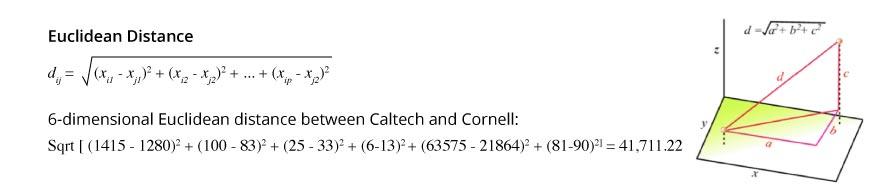In order to get a standardized distance, we have to normalize it.Hence standardized Euclidean distance between CalTech and Cornell are as follows: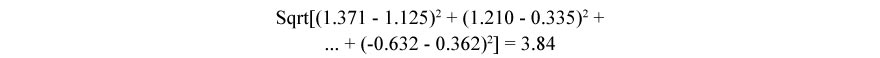{white} → {blue}

Support s = 4/10 = 0.4

Hence Support is 40%.

Support of a rule is defined as % (or number) of transactions in which antecedent (If) and consequent (Then) appear in the data.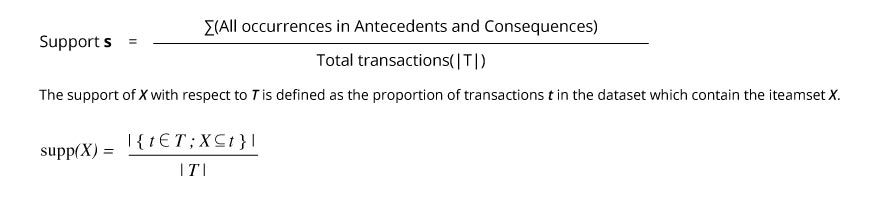{white} → {blue}

Confidence = 4 / 8

Confidence parameter is defined as: % of antecedent (If) transactions that also have the consequent (Then) itemset, same as P (Consequent | Antecedent) = P (C & A) / P (A)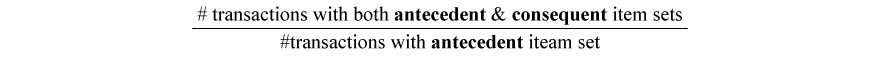{white} → {blue}

Lift = 0.4 / (0.5 * 0.8) = 0.4 / 0.4 = 1

Lift = confidence / (benchmark confidence)

Benchmark assumes independence between antecedent and consequent

P (Consequent & Antecedent) = P (C) * P (A)

Benchmark confidence

= P (C | A) = P (C & A) / P (A) = P (C) * P (A) / P (A)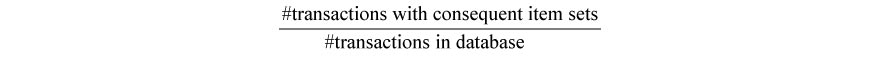Lift = Support (C U A) / [Support(C) * Support(A)]

Lift > 1 indicates a rule that is useful in finding consequent item sets (i.e. more useful than selecting transactions randomly)

## Description

Machine Learning is the field of study that provides the computers the capability to learn without being explicitly programmed. It is one of the most exciting technologies that one would have never come across. Machine Learning has become one of the most popular career choices today. According to a recent report from Gartner, Artificial Intelligence will create more than 2.3 million jobs by 2020.

A LinkdeIn study suggests that there are currently 1,829 jobs opening for  Machine Learning Engineering positions. Another study conducted by Analytical India Magazine reveals that there are more than 78,000 jobs in the Data Science and Machine Learning jobs lying across India. The demand for Machine Learning is growing at a faster pace. There are many factors contributing to increase in the demand of Machine Learning. Most companies are investing in machine learning. Companies are looking to hire more ML experts.

Jobs in machine learning rapidly increasing due to the increase in machine learning industry. The report from International Data Corporation estimates states that investing on Machine Learning and Artificial Intelligence will increase from $12B in 2017 to$57.6 B in 2021. Jobs in machine learning are highly paid since, the job is creative and unstructured, companies pay employees really well. The report from Glassdoor, states the average salary of machine learning engineers for freshers is between INR 4.5 lakhs to INR 7 lakhs, it might reach upto INR 16 lakhs for experienced professionals.

If you’re looking for interview questions and answers on machine learning for experienced and freshers, then you are at the right place. There are a lot of opportunities in many reputed companies across the globe. Good hands-on knowledge concepts will put you forward in the interview. You can find job opportunities everywhere. Our Machine Learning interview questions are exclusively designed for supporting employees in clearing interviews. We have tried to cover almost all the main topics related to Machine Learning.

Here, we have characterized the questions based on the level of expertise you’re looking for. Preparing for your interview with these interview questions on Machine Learning will give you an edge over other interviewees and will help you crack the Machine Learning interview easily. To get in-depth knowledge on Machine Learning you can also enroll for Machine Learning course.

All the best!